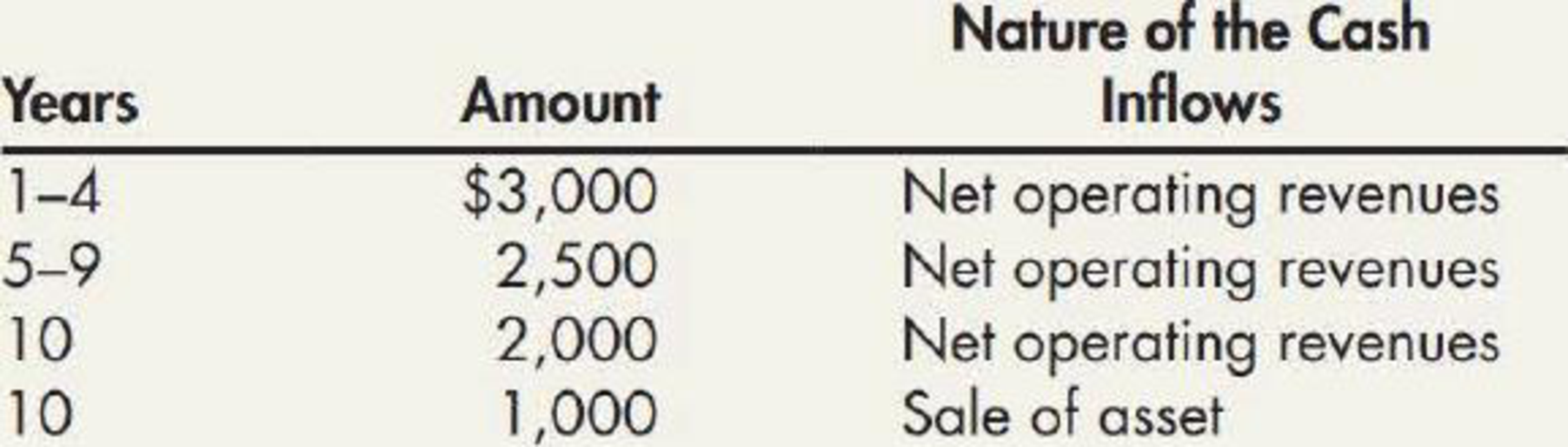Chapter M, Problem 19P### Intermediate Accounting: Reporting...

3rd Edition
James M. Wahlen + 2 others
ISBN: 9781337788281

#### Solutions

Chapter
Section### Intermediate Accounting: Reporting...

3rd Edition
James M. Wahlen + 2 others
ISBN: 9781337788281
Textbook Problem
1 views

# Asset Purchase Price BWP Inc. is considering the purchase of an asset. BWP’s required rate of return on new assets is 12%. The expected net cash inflows generated by the new asset are as follows:Required:Given that the net cash inflows can be realized, what is the maximum amount BWP should be willing to pay for the new asset? If BWP pays that amount, at what amount should BWP recognize the asset on the balance sheet? Assume that each cash inflow occurs at the end of the year. (Contributed by Norma C. Powell)

To determine

Determine the maximum amount that Incorporation B would be willing to pay for the new asset, and determine the amount at which the asset should be recorded on the balance sheet of Incorporation B.

Explanation

Present value:

The value of today’s amount to be paid or received in the future at a compound interest rate is called as present value.

 Years Net cash inflows (a) Present value factor @ 12% (b) Present values (c)=(a)×(b) 1-4 $3,000 3.037349$9,112.05 5-9 $2,500 (5.328250-3.037349)$5,727.25 10 \$2,000 0

### Still sussing out bartleby?

Check out a sample textbook solution.

See a sample solution

#### The Solution to Your Study Problems

Bartleby provides explanations to thousands of textbook problems written by our experts, many with advanced degrees!

Get Started

#### Find more solutions based on key concepts